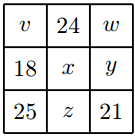Magic square

Algebra Level 2Shown right is a "magic square" in which the sums of each diagonal, each row, and each column are all equal.

Find $y+z.$

×